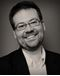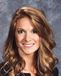# Grade 9 - 11 Algebra - Math

Sale ends in
Teacher: Anne
Customers Who Have Viewed This Course: 4694
\$279.00
\$128.00

#### 0 Final Exam Part I (Units 1-3) 00:00

This is an optional final exam to assess learning over units 1-3 as a whole. To grade this final, find the answer key in the resources section.

#### 0 Final Exam Part II (Units 4-6) 00:00

This is an optional final exam to assess learning over units 4-6 as a whole. To grade this final, find the answer key in the resources section.

Course Description

Math for 9th – 11th Grade weaves together previous study of Algebra concepts into a preparatory course for Algebra 2, Trigonometry, and Precalculus.  The course progresses from foundational concepts like understanding the real number system and conversion of units of measure to linear equations and inequalities before moving into a study of polynomials with an emphasis on quadratic equations.  It then extends into the complex number system and explores topics like vectors, matrices, and right triangle properties and trigonometry. The course topics address both abstract mathematical concepts as well as real-world problem situations, uniquely blending both algebraic and geometric concepts and approaches. There is consistent emphasis on explaining mathematical terminology, knowing that this is key for overcoming common stumbling blocks and reaching deeper understanding and therefore confidence. The course focuses on the mastery of critical and foundational skills and exposure to new skills necessary for success in subsequent math courses.

Course Includes

• 16+ hours of engaging instructional video
• 6 Units
• 50 video lessons
• 47 practice worksheets with printable answer keys
• 47 worksheet review videos where I go over each of the exercises
• 47 online “Knowledge Check” quizzes
• 15 “Challenge Problems” as extensions to each unit’s knowledge
• Solutions sets to each of the 47 “Knowledge Check Quizzes”
• Solutions sets to each of the 15 challenge problems
• Guided solutions showing step by step work to each of the 15 challenge problems
• Final Exam for Units 1-3
• Final Exam for Units 4-6
• Solutions sets for both final exams
• Introduction to Desmos video showing helpful hints for first time users

Course Goals

Upon completion of this course, students will be prepared to move on to math courses which demands strong algebra skills such as: Trigonometry, analytic Geometry, Precalculus, Linear Algebra, and eventually courses like Calculus and Statistics.

Course Requirements

Student taking this course are expected to have basic algebra skills such simplify expressions using order of operations, plotting points and graphing lines in slope-intercept form (y = mx + b) .

Target Audience

This video course is primarily intended for students who have already mastered Algebra 1 concepts and is designed so that it can be taken before or after both Geometry and Algebra 2. This course will also help students in building their mathematical and analytical skills which will prepare them for taking other courses in mathematics and the sciences.

Technology

Students will need a calculator capable of performing the basic operations, as well as square roots and trigonometric functions.

The majority of the lessons are taught so that technology is not needed. However there are some where the use of a calculator or computer is required. If the student has a scientific calculator (performs basic operations as well as square roots and trigonometric functions) or graphing calculator such as a TI-83 or 84 that will be sufficient, or a student can use the free online resources www.wolframalpha.com and www.desmos.com.

Under the Additional Resources for Parents are tutorial videos that show how to use either online resources or a calculator to perform the work needed.

Course Topics

Unit 1- Foundational Skills

Understand the Real Number System

Perform Arithmetic Operations on Rational Numbers

Rationalize the denominator

Understand the key components of graphing

Scaling graphs of data

Understand different measurement systems

Convert between units and systems of measure (Dimensional Analysis)

Solve application problems using measurement conversions

Area of 3D objects

Volume of 3D objects

Pythagorean Theorem

Unit 2- Linear Equations and Inequalities

Translate statements into mathematical expressions/equations/inequalities

Solve equations and inequalities with one variable

Substitution method to solve systems of linear equations

Elimination method to solve systems of linear equations

Graphing to solve systems of linear equations by graphing

Graphing systems of linear inequalities

Applications of systems

Unit 3- Polynomials

Factor polynomials using the GCF

Factor using the difference of two squares

Factor perfect square trinomials

Solve quadratic equations by square root method

Understand and apply the Zero Product Property

Solve quadratic equations by graphing, finding intersections

Long division of polynomials

Polynomial Remainder Theorem

Write polynomial equations from roots

Find real and non-real roots of polynomial equations

Key characteristics of polynomial equations

Graphing polynomial equations

Fundamental Theorem of Algebra

Unit 4- Complex Numbers

Understand the complex number system

Powers of i

Understanding the complex plane

Conjugates of complex numbers

Multiplying/Dividing complex numbers

Absolute Value of complex numbers

Graphing complex numbers

Unit 5- Vectors and Matrices

Understanding vectors v. scalars

Vector notation

Graphing vectors

Unit Vectors

Scalar multiplication on vectors

Magnitude of vectors

Matrix notation and dimensions

Scalar multiplication on matrices

Matrix multiplication

Zero Matrix

Identity Matrix

Determinant of a matrix

Inverse Matrices

Unit 6- Right Triangles

Converse of the Pythagorean Theorem

Classifying triangles by sides and angles

Right Triangle Trigonometry

Solving for Unknown Sides using trigonometry

Solving for unknown angles using trigonometry

Trigonometry applications

• Teacher: Anne
• Areas of expertise: Mathematics Grade 6-12
• Education: MAT, Wheaton College 2016; BS in Mathematics, Wheaton College 2011
• Interests: writing, sports, music, all sorts of board and card games
• Skills: play piano, video edit, coach basketball
• Issues I care about: Helping students become far more confident in mathematics than they thought was possible!

#### Test Preparation Document

Test Preparation Document

#### Digital Quizzes and Tests Answer Keys Document

Digital Quizzes and Tests Answer Keys Document

#### 1.1 Knowledge Check

These are the answer keys for both parts of the final. Use these to grade and score the exams.

#### Introduction to Desmos

Helpful hints for first time Desmos users!

#### Unit 1 Challenge Problems

These problems are designed to be an optional extension for students who are looking to challenge themselves using the knowledge gained from Unit 1.

#### Guided Solutions to Unit 1 Challenge Problems

These show a full step by step process with comments added in to help explain the work to solve each Challenge Problem.

#### Answer Key for Unit 1 Challenge Problems

These include only the answers in order to first check accuracy of your work.

#### Answers to Knowledge Check Quizzes

All of the answer keys to the online multiple choice quizzes called "Knowledge Checks" are included.

#### Unit 2 Challenge Problems

These problems are designed to be an optional extension for students who are looking to challenge themselves using the knowledge gained from Unit 2.

#### Guided Solutions to Unit 2 Challenge Problems

These show a full step by step process with comments added in to help explain the work to solve each Challenge Problem.

#### Answer Key for Unit 2 Challenge Problems

These include only the answers in order to first check accuracy of your work.

#### Unit 3 Challenge Problems

These problems are designed to be an optional extension for students who are looking to challenge themselves using the knowledge gained from Unit 3.

#### Guided Solutions to Unit 3 Challenge Problems

These show a full step by step process with comments added in to help explain the work to solve each Challenge Problem.

#### Answer Key for Unit 3 Challenge Problems

These include only the answers in order to first check accuracy of your work.

#### Unit 4 Challenge Problems

These problems are designed to be an optional extension for students who are looking to challenge themselves using the knowledge gained from Unit 4.

#### Guided Solutions to Unit 4 Challenge Problems

These show a full step by step process with comments added in to help explain the work to solve each Challenge Problem.

#### Answer Key for Unit 4 Challenge Problems

These include only the answers in order to first check accuracy of your work.

#### Unit 5 Challenge Problems

These problems are designed to be an optional extension for students who are looking to challenge themselves using the knowledge gained from Unit 5.

#### Guided Solutions to Unit 5 Challenge Problems

These show a full step by step process with comments added in to help explain the work to solve each Challenge Problem.

#### Unit 6 Challenge Problems

These problems are designed to be an optional extension for students who are looking to challenge themselves using the knowledge gained from Unit 6.

#### Guided Solutions to Unit 6 Challenge Problems

These show a full step by step process with comments added in to help explain the work to solve each Challenge Problem.

#### Answer Key for Unit 5 Challenge Problems

These include only the answers in order to first check accuracy of your work.

#### Answer Key for Unit 6 Challenge Problems

These include only the answers in order to first check accuracy of your work.
Customers who bought this item also boughtThis practical creative writing course will get students excited to create their own works of fiction, poetry, drama, and creative non-fiction. Writing techniques and skills will also be covered to help students discover and perfect their own writer's voice. +
\$299.00 \$137.00This course offers a full year of 7th and 8th grade science curriculum! Serving as an excellent precursor to high school biology, chemistry, and physics, it prepares students to tackle advanced science courses in high school while leaving them hungry to learn more! +
\$299.00 \$179.00This course will give you all the necessary skills needed in 5th grade math. You will find 40 video lessons with practice worksheets, practice tests and resources needed for you to be prepared for sixth grade. +
\$199.00 \$91.00